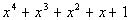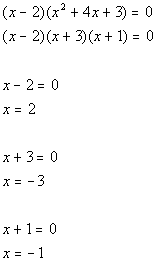# Polynomial

A polynomial is an expression with any number of terms that has real coefficients and has whole number exponents.

# Characteristics of polynomials

There are two ways to identify a polynomial. These include using the graph or the equation. While identifying a polynomial function you will find its degree, its end behavior, the number of extrema it has, its y-intercept, and its x-intercept.

### Degree

The degree of the polynomial function is the degree of the polynomial that has the largest power of the variable. So, to find the degree of a function you should look for the largest exponent in the function. When finding the degree of a function you should drop the coefficients or ignore them because they do not affect the degree.
Degrees
x —> linear
x2 —> quadractic
x3 —> cubic
x4 —> quartic
x5 —> quintic
x6 —> hecix
x7 —> heptic
x8 —> octic
x9 —> nonic
x10 —> decic
xn (n>10) —> nth degree

Examples:
f(x)=3x —> linear
f(x)=8x3 —> cubic
f(x)=7x7+5x6-4x5+8x+7 —> heptic
f(x)= 5x18-7x+9 —> 18th Degree

### Identifying Extrema

odd degree, m
Extrema: 0,2,…evens < m

even degree, m
Extrema: 1,3,…odds < m

Examples:
f(x)=3x5 —> extrema: 0,2,4
f(x)=7x8+3x+7 —> extrema: 1,3,5,7
f(x)=x2+5x+6 —> extrema: 1

### End Behavior

The end behavior of a polynomial function is the direction the graph is going as x reaches negative infinity and positive infinity.
To find the end behavior from an equation you're going to use two things to determine it. The two things you need to use is the degree of the function and the leading coefficient. The leading coefficient determines if the graph will be following a negative or positive path. The degree determines if its and even graph or odd graph.

 Degree Leading Coefficient End behavior Graph odd + ↓…↑(down up)odd - ↑…↓(up down)even + ↑…↑(up up)even - ↓…↓(down down)Examples:
f(x)=4x7-2x5+8x —> end behavior: ↓↑
f(x)=-8x-9 —> end behavior: ↑↓
f(x)=15x8+8x10+9x8-17x+23 —> end behavior: ↑↑

### Identifying the y-intercept and the x-intercepts

y-intercept
You can determine the y-intercept both of a graph and that of an equation. On a graph the point where the function crosses the y-axis is the y-intercept. Finding the y-intercept for a function is different. To find it in an equation you must plug zero in for the variable(s). The value that is leftover is the y-intercept.
Examples:
$y=13x+92$
$y=13(0)+92$
$y=92$

$y=2x^2+17x-4$
$y=2(0)^2+17(0)-4$
$y=-4$

x-intercepts
The x-intercepts of a function are also known as the roots or actors of a function. The amount of zeros and the method to determine the zeros of a function depends on the degree. to find the zeroes you can factor the equation or you can use quadratic formula if the equation isn't factorable. The Quadratic Formula is $x=\frac{-b\pm\sqrt{b^2-4ac}}{2a}$.

• Factor

$y=7x-49$
$49=7x$
$x=7$
7 is the zero of the function.
$y=x^2+2x-15$
$y=(x+5)(x-3)$
$x+5=0$ $x-3=0$
$x=-5$ $x=3$
-5 and the 3 are the zeros of the function.

• Quadratic Formuala

$x=\frac{-b\pm\sqrt{b^2-4(ac}}{2a}$
$x=\frac{-2\pm\sqrt{2^2-4(1)(-15)}}{2(1)}$
$x=\frac{-2\pm\sqrt{4-4(15)}}{2}$
$x=\frac{-2\pm\sqrt{4+60}}{2}$
$x=\frac{-2\pm\sqrt{64}}{2}$
$x=\frac{-2\pm8}{2}$
$x=-1\pm4$
$x=-1+4=3$ —> $x=3$
$x=-1-4=-5$ —> $x=-5$
3 and -5 are the zeros of the function.

The Zeros of a function can have multiplicity. This means there is more than one of the same zero. When this occurs it changes the way the curve crosses the x axis. If there o\is one multiplicity then it passes straight through the x-axis. If it is an even multiplicity the curve bounces off the x-intercept at the zero. If its an odd multiplicity it passes through the x-axis but with a wiggle, it doesn't go straight through.

# Factors and Polynomial Division

### How to Factor Polynomials

1. Greatest Common Factor(GCF)
Some polynomials made need to start of with simple factoring. The first thing to do when always factoring is take out the greatest common factor if there is one. The GCF is a number tht divides out all the leading coefficients or variables in the equation.
Equation GCF Leftover Factored polynomial
2x3+4x 2x x2+2 2x(x2+2)
9x4-18x2+12x+36 3 3x4-6x2+4x+12 3(3x4-6x2+4x+12)
4x4y3+64x2y-12x3y8^^ 4x2y x2y2+16-3xy7 4x2y(x2y2+16-3xy7)

2. Factoring Trinomials.
When you factor you want to "unfoil" the equation. Just remember when we factor we always take out the GCF if there is one. When factoring we look as the leading coefficient and the constant, or a and c in $y=ax^2+bx+c$. We look at their possible factors. these possible factors have to multiplied by each other to get the leading coefficient, when looking at a, and the constant, when your looking a c. These factor of both when multiplied and added together must equal b.

Equation factors of leading coefficient factors of constant factored equation
y=x2+x3+2 x and x 2 and 1 (x+2)(x+1)
y=x^^-3x-10 x and x -5 and 2 (x-5)(x+2)

### Synthetic Division

Synthetic division is used when dividing a polynomial by a binomial. The binomial is in the form $x-c$, where c is the constant. Synthetic division can also be used in finding factors and zeros. The Factor Theorem stays, if the functional value is 0 at some value c, then x - c is a factor and c is a zero.
Step 1: set up the synthetic division
The divisor (the number you are dividing by) goes on the outside of the box, while the dividend (the number you are dividing into) goes on the inside of the box. Write the dividend in descending powers, and for missing term place in 0.Step 2: Bring the leading coefficient down below the box.Step 3: Multiple the constant, c, to the value just added to the bottom of the box and plac the product under the next dividendStep 4: Now with the second column made add the two values togetherStep 5: Repeat until the last variable inside the boxStep 6: Write out the answer
The numbers in the bottom row make up the coefficients of the new quotient as well as the remainder. The last value on the right is the remainder.###### Synthetic Division to find zeros of the function
You will use synthetic the division the same exact way. The difference is what final answer is going to be. Now, we are looking for all the zeros of the function. We will start by dividing using synthetic division and then rewrite f(x) as (x - c)(quotient).Notice how the remainder is 0. This only works when the remainder is 0. This means that (x - 2) is a factor $f(x)=x^3+2x^2-5x-6$.The zeros of this function are x = 2, -3, and -1.

# Graphing Polynomial Functions

Steps to graphing a polynomial function:

1. Determine the x-intercepts and their multiplicity —> this can be done by factoring, using quadratic formula, or using synthetic division to find the zeros of the function.
2. Determine the y-intercept —> this can be done by setting the variables in the equation to zero and solving for y.
3. Find the end behavior of the graph —> this can be done by looking at the of the leading coefficient, to determine if the graph is positive or negative, and the power of the leading coefficient, to determine the degree of the function.
4. Plot the zeros on the graph
5. Plot the y-intercept on the graph
6. Now using the end behavior start sketching. Sketch through the x-intercepts or bounce of them depending on the multiplicity of the x-intercept. Pass the curve through the y-intercept too. The end behavior will tell you if you have to start down and go either up or down, or it will tell you start up and go down or up.
Example:
Graph:Find the zeros:So from this we know x=-1 will cause the curve to bounce ther and x=2 will cause the curve to cross the x-axis, but with a wiggle.
Find the y-intercept:End bahvior:
The coefficient is to the 5th degree term is positive, because degree is odd we know that this polynomial will start down at the right end and end up at the left. If you feel like it you could plug in some values in the equation to get pints on the grpah to put on the graph
*OptionalThis is the sketch